##### Common Core Math For Parents For Dummies with Videos Online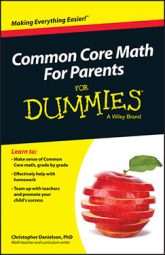In Common Core math, sixth grade students study ratios and fractions that are set in understandable contexts, and they work them in order to ask and answer interesting and challenging questions.

A ratio is a comparison of two numbers that depends on the multiplication relationship between them. Here are some examples of ratios:

• A recipe for orange juice calls for 3 cans of cold water for each 1 can of frozen concentrate.

• The city of Saint Paul requires 5 parking spaces for every 1,000 square feet of retail space in a new development.

• A certain shade of green paint requires 3 parts of blue paint for every 2 parts of yellow paint.

You can write a ratio in three different ways: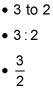In each case, you can read the ratio as three to two. Sixth graders typically work with the first two of these forms rather than the fraction form because of important, but subtle, differences between fractions and ratios.

You can think of a fraction as a number. The fraction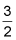has a place on the number line — halfway between 1 and 2, as shown in the figure.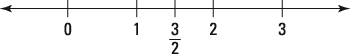A fraction is a number on the number line.

A ratio, though, isn't a number. It's a comparison of two numbers. A ratio doesn't belong on the number line. The notations 3 to 2 and 3:2 can help students remember that a ratio isn't a number.

The reason the fraction form exists is that in some ways, ratios behave exactly like fractions. Think about the ratio in a recipe for green paint: 3 parts of blue paint to 2 parts of yellow paint. If you make that recipe twice, your ratio will be 6 parts blue paint to 4 parts yellow paint, and you'll still have the same color of green. In that way, 3:2 is the same as 6:4.

Working with fractions is identical. The fractionis equivalent to the fraction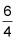because they occupy the same location on the number line.

You can write equivalent ratios in the same way that you write equivalent fractions, which makes the fraction notation useful.